### IMO Shortlist 2006 problem C3

Kvaliteta:
Avg: 0,0
Težina:
Avg: 7,0
Let$S$ be a finite set of points in the plane such that no three of them are on a line. For each convex polygon$P$ whose vertices are in$S$, let$a(P)$ be the number of vertices of$P$, and let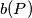$b(P)$ be the number of points of$S$ which are outside$P$. A line segment, a point, and the empty set are considered as convex polygons of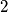$2$,$1$, and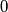$0$ vertices respectively. Prove that for every real number$x$:$\sum_{P}{x^{a(P)}(1 - x)^{b(P)}} = 1$, where the sum is taken over all convex polygons with vertices in$S$.

Alternative formulation:

Let$M$ be a finite point set in the plane and no three points are collinear. A subset$A$ of$M$ will be called round if its elements is the set of vertices of a convex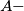$A -$gon$V(A).$ For each round subset let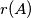$r(A)$ be the number of points from$M$ which are exterior from the convex$A -$gon$V(A).$ Subsets with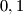$0,1$ and 2 elements are always round, its corresponding polygons are the empty set, a point or a segment, respectively (for which all other points that are not vertices of the polygon are exterior). For each round subset$A$ of$M$ construct the polynomialShow that the sum of polynomials for all round subsets is exactly the polynomial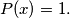$P(x) = 1.$
Izvor: Međunarodna matematička olimpijada, shortlist 2006